Domain & Range

Domain & Range

 Site: TBAISD Moodle Course: Michigan Algebra I Preview 2012 Book: Domain & Range Printed by: Guest user Date: Friday, April 16, 2021, 10:28 PM

Introduction

As discussed previously, for exponential functions of the form,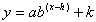, there is no restriction on the value of x. Therefore, the function is defined for all real values of x, and the domain is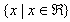.

All exponential functions have an asymptote, or line that the graph will approach but never touch or cross. Therefore, the range of an exponential function of the form,, is dependent on the sign of a and the value of the asymptote. If a is a positive value then the graph will approach the asymptote from the top, which means the range will be greater than the asymptote. If a is negative, then the graph will approach the asymptote from the bottom, which means the range will be less than the asymptote. Because the graph never intersects the asymptote, the range will never include this value.

Listen

Example 1

Find the domain and range of the function: g(x) = 3.2x+1 + 2.

Step 1. Find the domain.

Since the function is not representing a situation, the x can be any real number.

Therefore, the domain is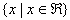.

Step 2. Find the range.

This graph is shifted up 2 and has an asymptote at the line y = 2. It also has a positive a-value.

Therefore, the range of the function is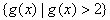.

Example 2

Find the domain and range of the function: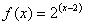.

Step 1. Find the domain.

Since the function is not representing a situation, the x can be any real number.

Therefore, the domain is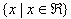.

Step 2. Find the range.

This graph is shifted to the right 2, which does not affect the asymptote. It has an asymptote at y = 0. It also has a positive a-value.

Therefore, the range of the function is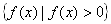.

Example 3

Find the domain and range of the function: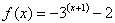.

Step 1. Find the domain.

Since the function is not representing a situation, the x can be any real number.

Therefore, the domain is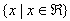.

Step 2. Find the range.

This graph is shifted down 2 and has an asymptote at y = -2. It also has a negative a-value.

Therefore, the range of the function is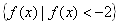.

Listen

From a Graph

To find domain and range of an exponential function by looking at a graph, determine where the x and y-values of the graph are bounded. For domain, look at the graph and find what values of x will be included in the graph. For range, look at the graph and find what values of y are included in the graph.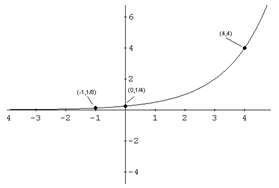In the graph above, since all x values appear to be present in the graph, the domain is all real numbers or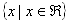. Since the horizontal asymptote is y = 0, there will not be any values below, or at, y = 0. The range is y > 0, or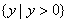.

Listen

Example

Find the domain and range of the graph below.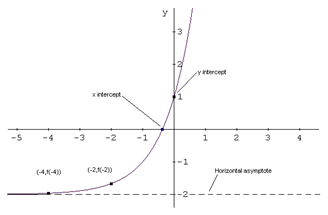Step 1. Find the domain.

Since all x values appear to be present in the graph, the domain is all real numbers, or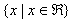.

Step 2. Find the range.

Since the horizontal asymptote is y = -2, then there will not be any values below, or at, y = -2. The range is y > -2, or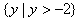.

Video Lesson

To learn how to determine the domain and range of an exponential function, select the following link:

Practice

Domain & Range of Exponentials Worksheet

Answer Key

Domain & Range of Exponentials Answer Key

Sources

Adams, R., & Free High School Science Texts.2009. Exponential Functions and Graphs - Grade 11. Connexions, July 29, 2009. http://cnx.org/content/m30856/1.1/.

Dendane, Dr Abdelkader, Ph.D. "Graph of Exponential Functions." November 25, 2007.http://www.analyzemath.com/Graphing/GraphExponentialFunction.html

Khan, Salman. "Identifying Exponential Models."

http://www.khanacademy.org/video/identifying-exponential-models (accessed September 11, 2010).